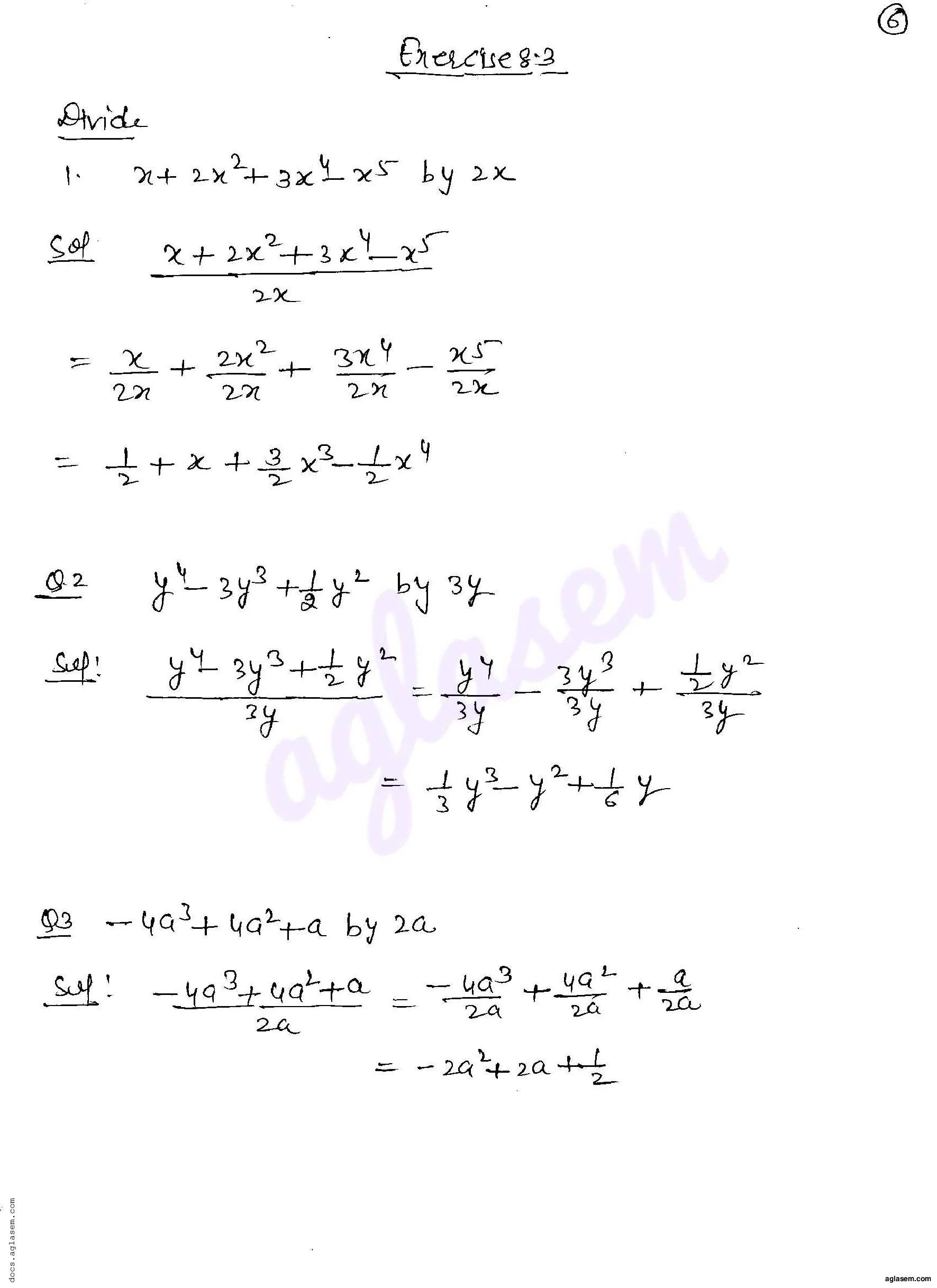# RD Sharma Solutions Class 8 Chapter 8 Division of Algebraic Expressions Exercise 8.3

Here you can get free RD Sharma Solutions for Class 8 Maths Chapter 8 Division of Algebraic Expressions Exercise 8.3. All RD Sharma Book Solutions are given here exercise wise for the chapter Division of Algebraic Expressions. RD Sharma Solutions are helpful in the preparation of several school level, graduate and undergraduate level competitive exams. Practicing questions from RD Sharma Mathematics Solutions for Class 8 Chapter 8 Division of Algebraic Expressions is proven to enhance your math skills.

 Class: Class 8th Chapter: Chapter 8 Exercise: Exercise 8.3 Name: Division of Algebraic Expressions

## RD Sharma Solutions Class 8 Chapter 8 Division of Algebraic Expressions Exercise 8.3

RD Sharma Class 8 Solutions Chapter 8 for Division of Algebraic Expressions Exercise 8.3 are given below.

RD Sharma Solutions Class 8 Chapter 8 Division of Algebraic Expressions Exercise 8.3RD Sharma Solutions for Class 8 Maths PDF download for Chapter 8 Division of Algebraic Expressions Exercise 8.3 is available here for free.

 « Previous Next »

### RD Sharma Solutions Class 8 Chapter 8 Exercise Wise

You can also see RD Sharma Solutions of all exercises of Chapter 8 – Division of Algebraic Expressions from here.

### RD Sharma Solutions Class 8 Chapter Wise

If you want RD Sharma solutions of any topic other than Chapter 8 Division of Algebraic Expressions, check it from here. Mathematics by RD Sharma has all chapters that are in your Class 8 syllabus.

Click to rate this post!
[Total: 2 Average: 5]